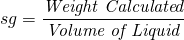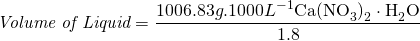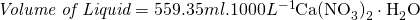Liquid fertilizers are treated slightly different than granular fertilizers. They do not have impurities, but their density or specific gravity need to be taken into account. In most calculations, it is assumed that fertilizers are granular, so we use the weight of the fertilizers. If it is a liquid, we would have used volumes such as milli-litres or litres. However, all calculations are done as if the fertilizers are granular, this makes the calculation easier and less prone to mistakes by mixing units of measure. It is only at the end of the calculation that the fertilizer amount is adjusted. For liquid fertilizers the density (D) or specific gravity (sg) is used to adjust the amount to be used. The specific gravity of water is 1. The specific gravity (sg) of the liquid fertilizer should be provided by the fertilizer provider.Equation to calculate the weight of a fertilizer if the volume and density or specific gravity are knownIn the example in the previous section, calcium nitrate was used and assumed it was a granular fertilizer. However, calcium nitrate can also be bought as a liquid. If it was a liquid, the final amount of fertilizer used had to be adjusted to compensate for its density or specific gravity. If we assume a specific gravity (sg) of calcium nitrate to be 1.8, the total adjusted amount of calcium nitrate that must be added can be calculated as follows:Thus 559 ml of calcium nitrate liquid must be added to 1000 L of water to provide 119.41 g.1000L-1 nitrogen and 170.66 g.1000 L-1 calcium.